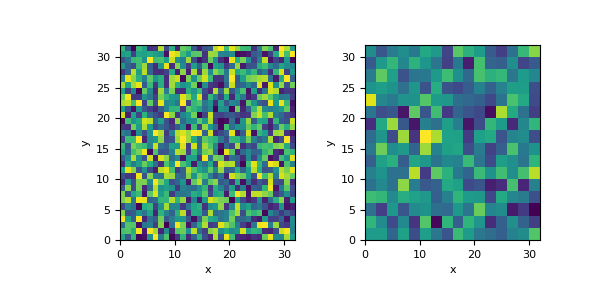# discretize.utils.volume_average¶

discretize.utils.volume_average(mesh_in, mesh_out, values=None, output=None)[source]

Volume averaging interpolation between meshes.

This volume averaging function looks for overlapping cells in each mesh, and weights the output values by the partial volume ratio of the overlapping input cells. The volume average operation should result in an output such that `np.sum(mesh_in.cell_volumes*values)` = `np.sum(mesh_out.cell_volumes*output)`, when the input and output meshes have the exact same extent. When the output mesh extent goes beyond the input mesh, it is assumed to have constant values in that direction. When the output mesh extent is smaller than the input mesh, only the overlapping extent of the input mesh contributes to the output.

This function operates in three different modes. If only mesh_in and mesh_out are given, the returned value is a `scipy.sparse.csr_matrix` that represents this operation (so it could potentially be applied repeatedly). If values is given, the volume averaging is performed right away (without internally forming the matrix) and the returned value is the result of this. If output is given as well, it will be filled with the values of the operation and then returned (assuming it has the correct `dtype`).

Parameters
mesh_in

Input mesh (the mesh you are interpolating from)

mesh_out

Output mesh (the mesh you are interpolating to)

values(`mesh_in.n_cells`) `numpy.ndarray`, `optional`

Array with values defined at the cells of `mesh_in`

output(`mesh_out.n_cells`) `numpy.ndarray` `of` `float`, `optional`

Output array to be overwritten

Returns
(`mesh_out.n_cells`, `mesh_in.n_cells`) `scipy.sparse.csr_matrix` `or` (`mesh_out.n_cells`) `numpy.ndarray`

If values = None , the returned value is a matrix representing this operation, otherwise it is a `numpy.ndarray` of the result of the operation.

Examples

Create two meshes with the same extent, but different divisions (the meshes do not have to be the same extent).

```>>> import numpy as np
>>> from discretize import TensorMesh
>>> h1 = np.ones(32)
>>> h2 = np.ones(16)*2
>>> mesh_in = TensorMesh([h1, h1])
>>> mesh_out = TensorMesh([h2, h2])
```

Create a random model defined on the input mesh, and use volume averaging to interpolate it to the output mesh.

```>>> from discretize.utils import volume_average
>>> model1 = np.random.rand(mesh_in.nC)
>>> model2 = volume_average(mesh_in, mesh_out, model1)
```

Because these two meshes’ cells are perfectly aligned, but the output mesh has 1 cell for each 4 of the input cells, this operation should effectively look like averaging each of those cells values

```>>> import matplotlib.pyplot as plt
>>> plt.figure(figsize=(6, 3))
>>> ax1 = plt.subplot(121)
>>> mesh_in.plot_image(model1, ax=ax1)
>>> ax2 = plt.subplot(122)
>>> mesh_out.plot_image(model2, ax=ax2)
>>> plt.show()
```